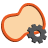Back to Adaptive Vision Library website

You are here: Start » Function Reference » Region Features

Region Features

Select a function from the list below.

IconNameDescriptionRegionAreaComputes the number of pixels contained in a region.RegionBoundingBoxComputes the smallest box containing a region.RegionBoundingBox_OrNilComputes the smallest box containing a region; returns NIL if the region is empty.RegionBoundingCircleComputes the smallest circle enclosing a region.RegionBoundingCircle_OrNilComputes the smallest circle enclosing a region; returns NIL if the region is empty.RegionBoundingParallelogramComputes the smallest parallelogram containing a region.RegionBoundingRectangleComputes the smallest rectangle containing a region.RegionBoundingRectangle_FixedAngleComputes the smallest rectangle with the given orientation angle containing a region.RegionBoundingRectangle_FixedAngle_OrNilComputes the smallest rectangle with the given orientation angle containing a region; returns NIL if the region is empty.RegionBoundingRectangle_OrNilComputes the smallest rectangle containing a region; returns NIL if the region is empty.RegionCaliperDiameterComputes the longest and the shortest width of the input region measured as distance between parallel lines containing the whole region.RegionCircularityComputes the area of a region divided by the area of a circular region having the same feature.RegionContoursComputes an array of closed paths corresponding to the contours of the input region.RegionConvexityComputes the area of a region divided by area of its convex hull.RegionDiameterComputes the longest segment connecting two pixels contained in region and its length.RegionEllipticAxesComputes axes of an ellipse having the same first and second order moments as the given region.RegionElongationComputes the elongation factor of a region ( perfect circle has minimal elongation equal 1.0 ).RegionHolesCreates regions of the holes of the input region.RegionInscribedBoxComputes the largest box contained in a region.RegionInscribedCircleComputes the largest circle contained in a region.RegionMassCenterComputes a point with coordinates equal to the average coordinates of the region's pixels.RegionMassCenter_OrNilComputes a point with coordinates equal to the average coordinates of the region's pixels; returns NIL if the region is empty.RegionMedialAxisComputes an array of paths corresponding to the skeleton of the input region.RegionMomentComputes selected second-order moment of a region in regular and normalized ( divided by region area ) variant.RegionNumberOfHolesComputes the number of holes in a region.RegionOrientationComputes the orientation of a region as an angle of value in range 0.0 - 180.0.RegionPerimeterLengthComputes the length of the input region perimeter.RegionPointRunLengthsReturns the lengths of horizontal sequences of locations that constitute the input region.RegionProjectionComputes the profile of the region pixel count in consecutive rows or columns.RegionRectangularityComputes the area of a region divided by the area of its bounding rectangle.